Check Important Questions and Chapter Summary -https://you.tube/Real-Numbers-Class-10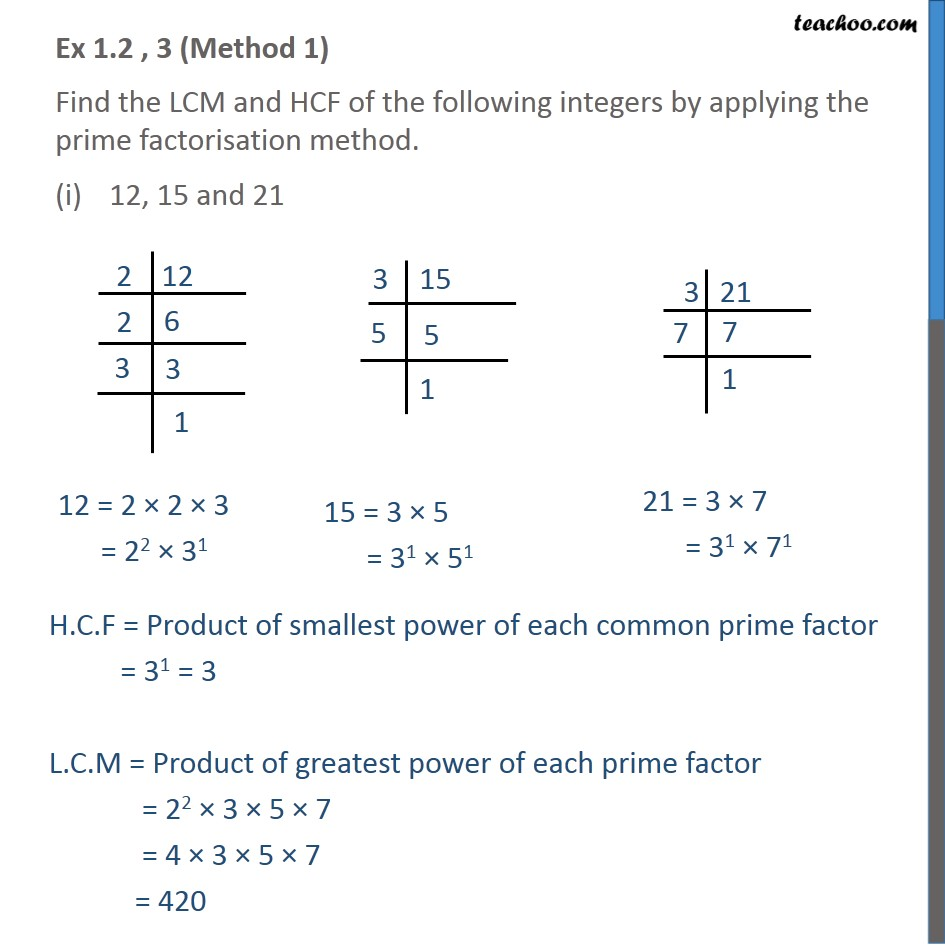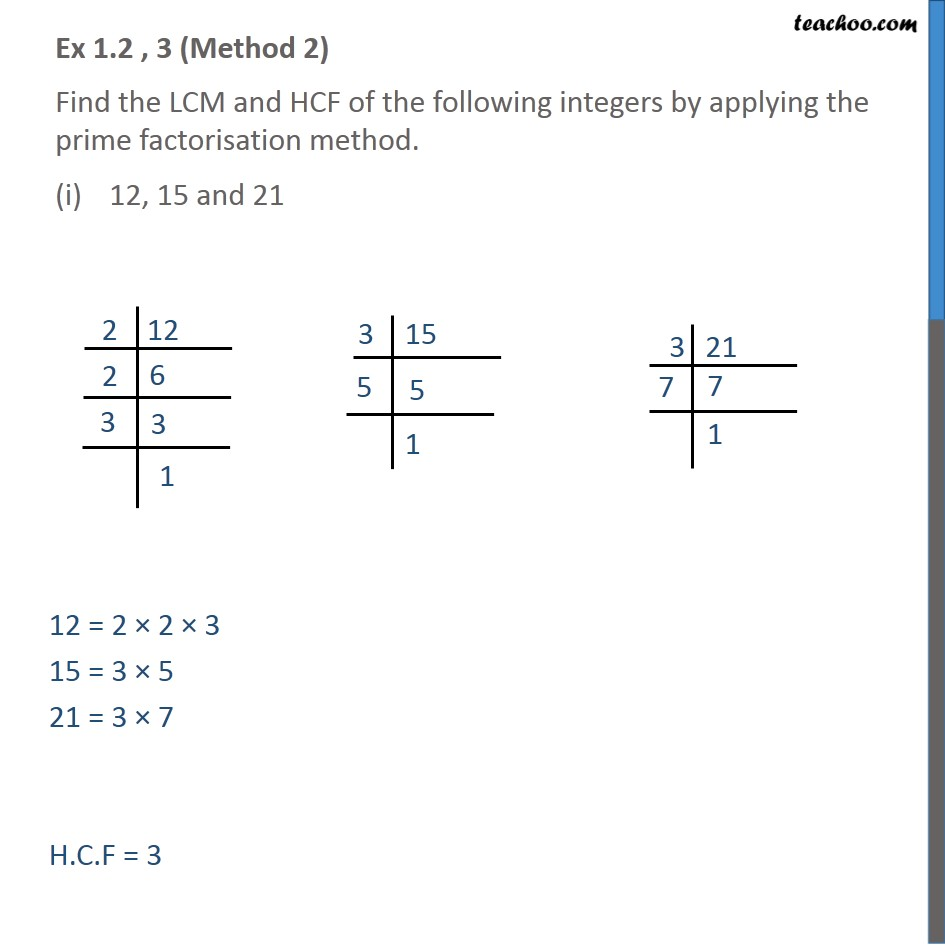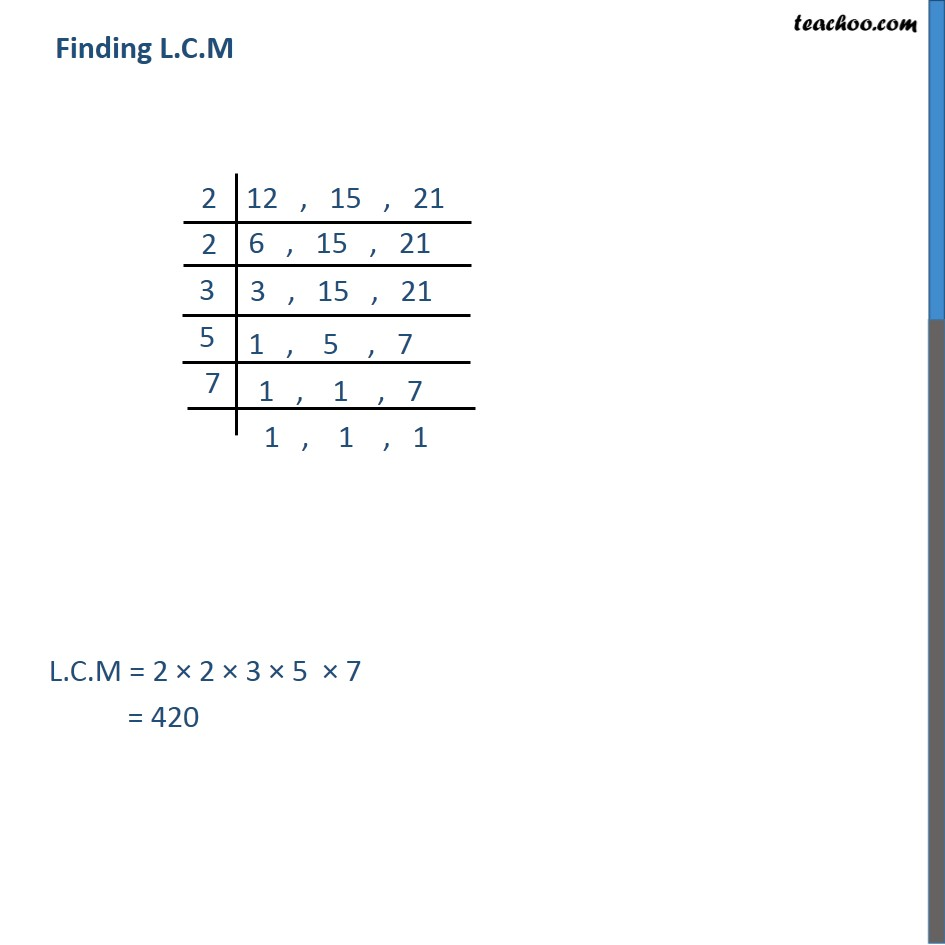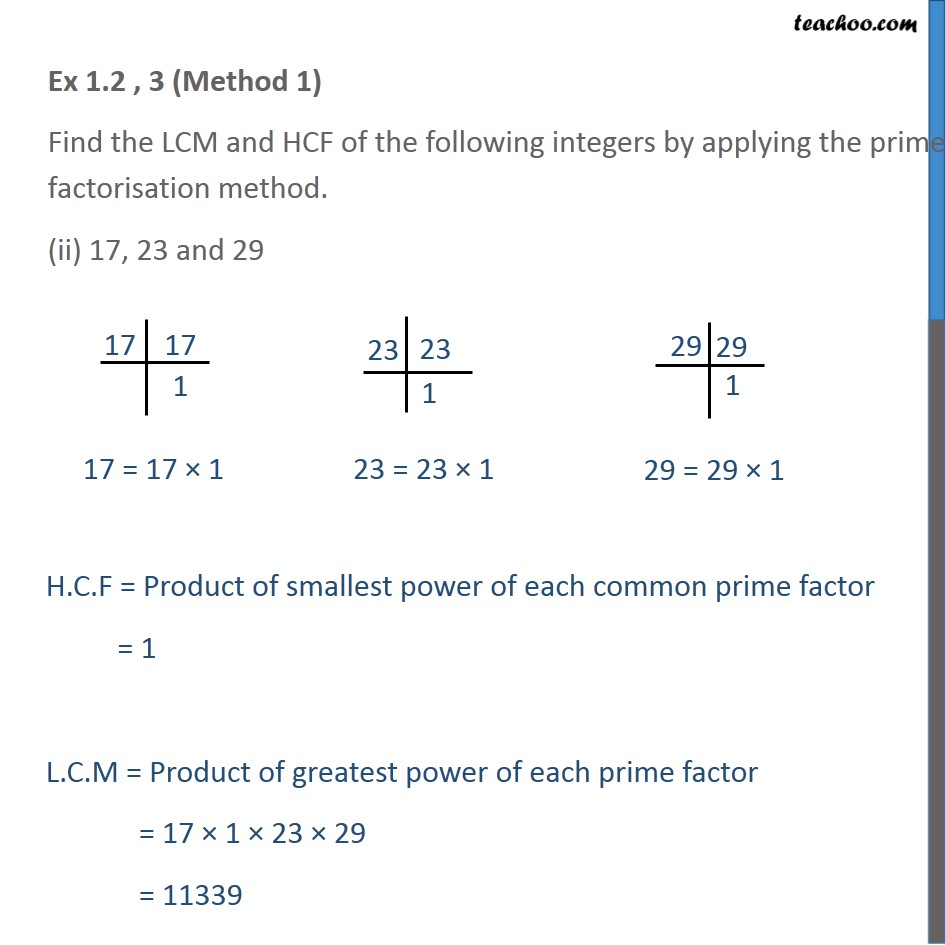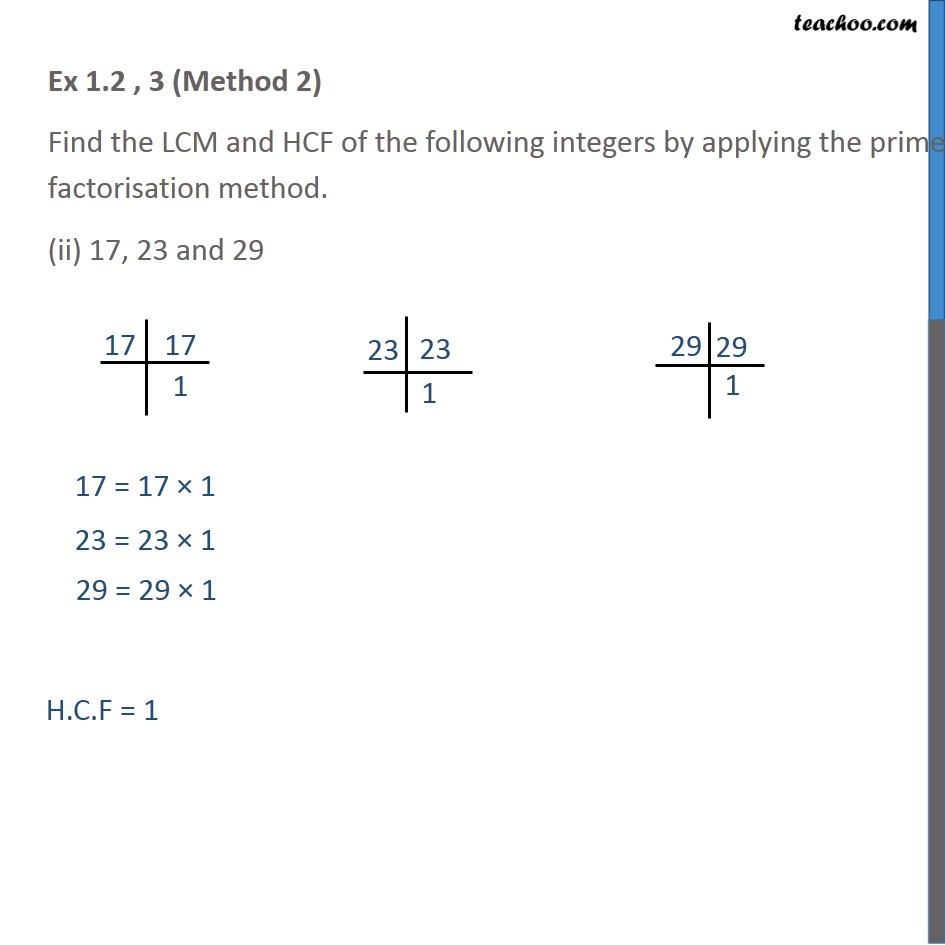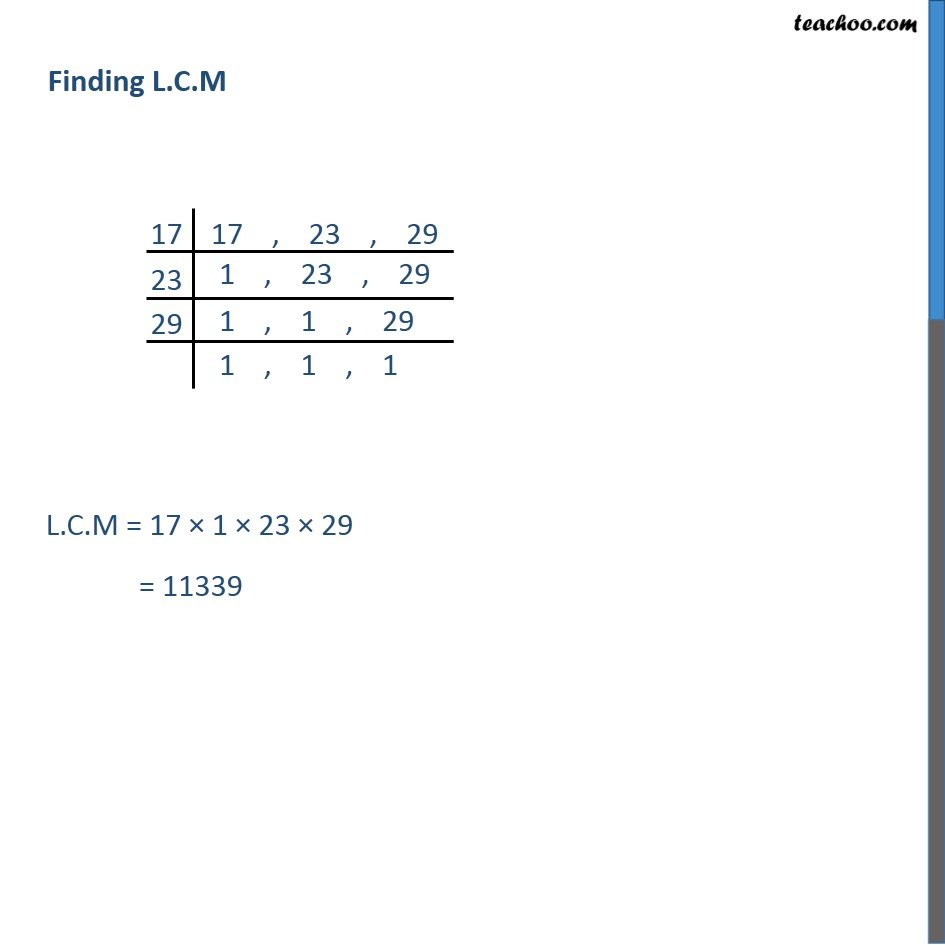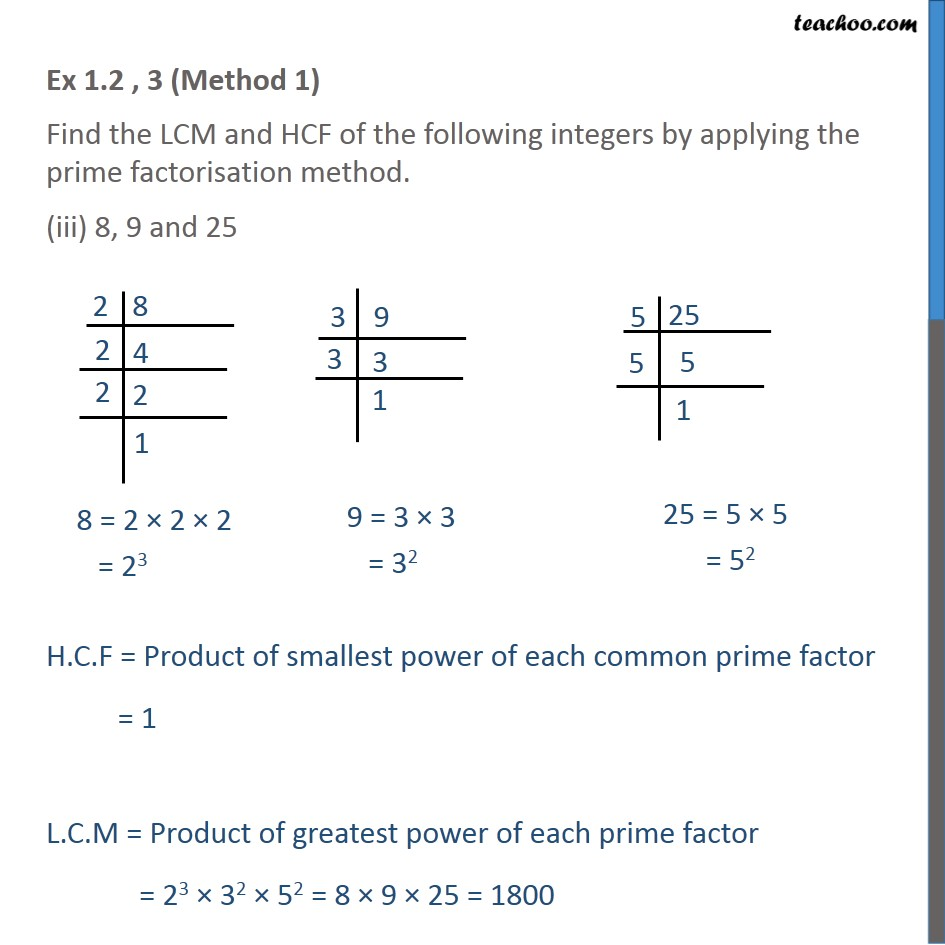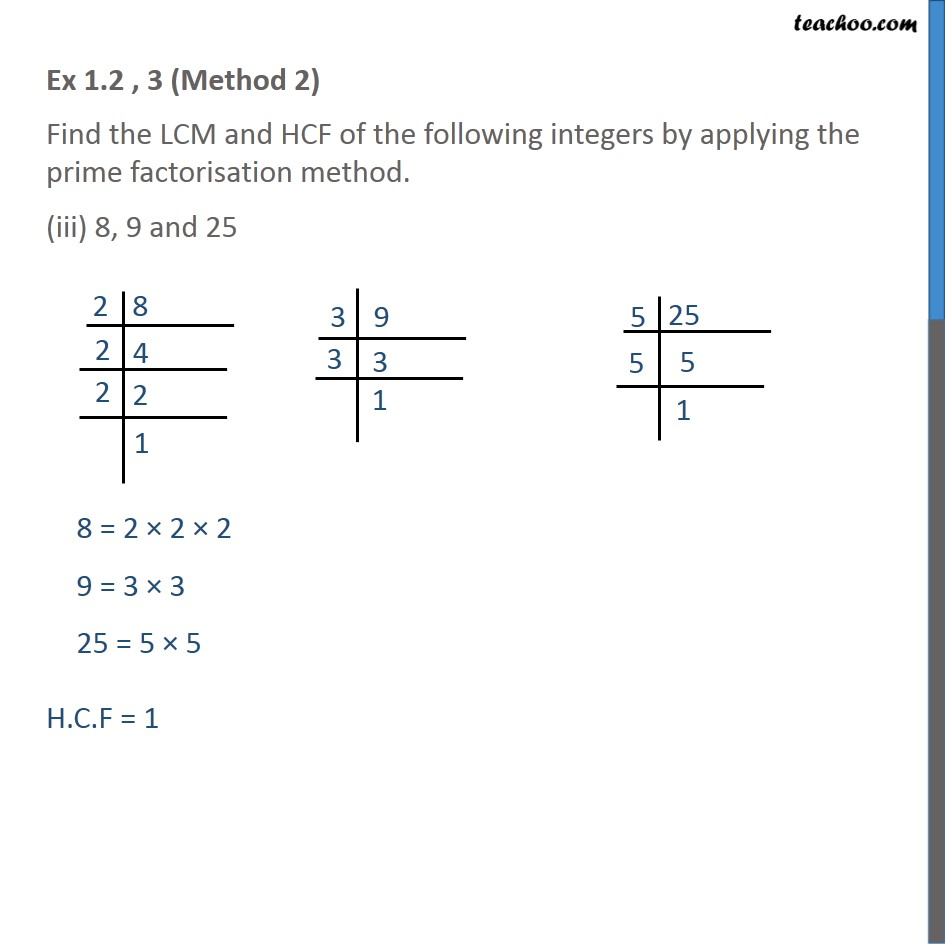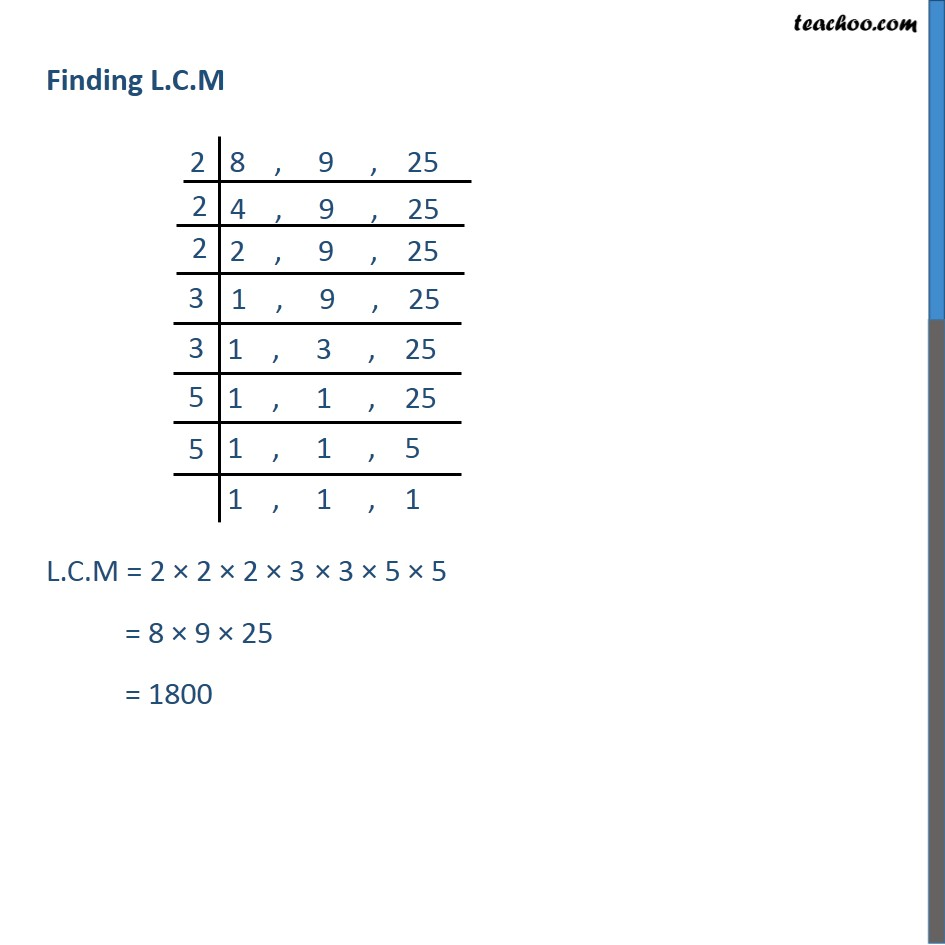1. Chapter 1 Class 10 Real Numbers
2. Serial order wise
3. Ex 1.2

Transcript

Ex 1.2 , 3 (Method 1) Find the LCM and HCF of the following integers by applying the prime factorisation method. 12, 15 and 21 Ex 1.2 , 3 (Method 2) Find the LCM and HCF of the following integers by applying the prime factorisation method. 12, 15 and 21 Finding L.C.M Ex 1.2 , 3 (Method 1) Find the LCM and HCF of the following integers by applying the prime factorisation method. (ii) 17, 23 and 29 Ex 1.2 , 3 (Method 2) Find the LCM and HCF of the following integers by applying the prime factorisation method. (ii) 17, 23 and 29 Finding L.C.M Ex 1.2 , 3 (Method 1) Find the LCM and HCF of the following integers by applying the prime factorisation method. (iii) 8, 9 and 25 H.C.F = Product of smallest power of each common prime factor = 1 L.C.M = Product of greatest power of each prime factor = 23 32 52 = 8 9 25 = 1800 Ex 1.2 , 3 (Method 2) Find the LCM and HCF of the following integers by applying the prime factorisation method. (iii) 8, 9 and 25 H.C.F = 1 Finding L.C.M L.C.M = 2 2 2 3 3 5 5 = 8 9 25 = 1800

Ex 1.2